# Brauer-Severi variety

(diff) ← Older revision | Latest revision (diff) | Newer revision → (diff)

An algebraic variety over a fieldthat, if considered over the algebraic closureof, becomes isomorphic to a projective space.

The arithmetic properties of such varieties were studied in 1932 by F. Severi; F. Châtelet subsequently discovered a connection between Brauer–Severi varieties and central simple algebras (cf. Central simple algebra) overand the Brauer group.

The simplest non-trivial example of a one-dimensional Brauer–Severi variety is the projective conic section:on the real projective plane. Over the field of complex numbersthis variety is isomorphic to the projective line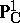. The set of all one-dimensional Brauer–Severi varieties, considered up to isomorphism, is in a one-to-one correspondence with the set of projective non-degenerate conic sections (considered up to projective equivalence over), which is in turn in a one-to-one correspondence with the set of non-isomorphic generalized quaternion algebras over. In the above example the conical section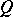corresponds to the algebra of ordinary quaternions.

In the more-dimensional case, the set of classes of-dimensional Brauer–Severi varieties (i.e. Brauer–Severi varieties distinguished up to-isomorphism) may be identified with the Galois cohomology groupwhere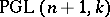is the projective group of automorphisms of the projective space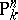, . This cohomology group describes the classes of-isomorphic central simple-algebras of rank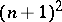(i.e. forms of the matrix algebra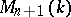). The connection between Brauer–Severi varieties and central simple algebras is more explicitly described as follows. To a-algebraof rank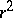one associates the varietyof its left ideals of rank, which is defined as a closed subvariety of the Grassmann manifold of all-linear subspaces of dimensionin. In certain cases the varietymay be defined by norm equations — e.g. in the case of quaternion algebras. The connection between Brauer–Severi varieties and algebras is taken advantage of in the study of both , .

The most significant properties of Brauer–Severi varieties are the following. A Brauer–Severi variety is-isomorphic to a projective space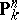if and only if it has a point in the field. All Brauer–Severi varieties have a point in some finite separable extensionof.

The Hasse principle applies to Brauer–Severi varieties defined over an algebraic number field.

The field of rational functions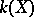on a Brauer–Severi varietyis the splitting field of the corresponding algebra; moreover, an arbitrary extensionofis the splitting field forif and only ifhas a-point .

In the context of the generalization of the concepts of a central simple algebra and the Brauer group to include schemes, the Brauer–Severi varieties were generalized to the concept of Brauer–Severi schemes . Letbe a morphism of schemes. A scheme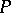is called a Brauer–Severi scheme if it is locally isomorphic to a projective spaceoverin the étale topology of. A schemeover a schemeis a Brauer–Severi scheme if and only if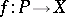is a finitely-presented proper flat morphism and if all of its geometrical fibres are isomorphic to projective spaces .